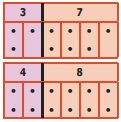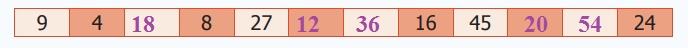Home | | Maths 6th Std | Numbers

# Numbers

Learning Objectives * To identify prime and composite numbers. * To know the divisibility rules and use them to find the factors of a number. * To write a composite number as a product of prime numbers. * To find the HCF and the LCM of two or more numbers and use them in real life situations.

CHAPTER 1

NUMBERS

Learning Objectives

* To identify prime and composite numbers.

* To know the divisibility rules and use them to find the factors of a number.

* To write a composite number as a product of prime numbers.

* To find the HCF and the LCM of two or more numbers and use them in real life situations.

Recap

1. Odd and Even Numbers

* A number is called an odd number if it cannot be grouped equally in twos. 1, 3, 5, 7, …, 73, 75, …, 2009,… are odd numbers.

* A number is called an even number if it can be grouped equally in twos. 2, 4, 6, 8, ..., 68, 70, . . , 4592,... are even numbers.* All odd numbers end with anyone of the digits 1, 3, 5, 7 or 9.

* All even numbers end with anyone of the digits 0, 2, 4, 6 or 8.

* In whole numbers, odd and even numbers come alternatively.

Try these

(i) Observe and complete:

1+3 = ?

5+11 = ?

21+47 = ?

........+....... = ?

1 + 3 = 4

5 + 11 = 16

21 + 47 = 68

33 + 51 = 84

From this observation, we conclude that “the sum of any two odd numbers is always an even number

(ii) Observe and complete:

5 × 3 = ?

7 × 9 = ?

11 × 13 = ?

.... × ..... = ?

5 × 3 = 15

7 × 9 = 63

11 × 13 = 143

23 × 15 = 345

From this observation, we conclude that “the product of any two odd numbers is always an odd number

Justify the following statements with appropriate examples:

(iii) The sum of an odd and an even number is always an odd number.

(iv) The product of an odd and an even number is always an even number.

(v) The product of any three odd numbers is always an odd number.

Note

* 1 is odd, its successor 2 is even and so its predecessor 0 is also even.

* The first natural number 1 is odd and the first whole number 0 is even.

2. Factors

The teacher gives Velavan two numbers 8 and 20 and asks him to write them as a product of two numbers. Velavan, with his mental math skills and also using the multiplication tables, quickly finds that 8 = 2 × 4; 20 = 2 × 10 and 4 × 5. From this, we can say that 2 and 4 are factors of 8 and also 2, 4, 5 and 10 are factors of 20. We can also write 8 as 1 × 8 and hence conclude that 1 and 8 are also factors of 8.

From the above examples, we observe that,

* A factor is a number that divides the given number exactly (gives remainder zero).

* Every number has two factors that is 1 and the number itself.

* Every factor of a number is less than or equal to that number.

3. Multiples

Look at the multiplication table of (say) 7:

1 × 7 = 7

2 × 7 = 14

3 × 7 = 21

4 × 7 = 28

5 × 7 = 35 ...

We say that the numbers 7, 14, 21, 28, 35,... are multiples of 7.

From this, we observe that

* Every multiple of a number is greater than or equal to that number.

Multiples of 7 are 7, 14, 21, 28,... . They are greater than or equal to 7.

* Multiples of a number are endless.

Multiples of 5 are 5, 10, 15, 20, . They are endless.

Try these

(i) Say True or False.

a) The smallest odd natural number is 1. [True]

b) 2 is the smallest even whole number. [False]

( The first whole number is o)

c) 12345 + 5063 is an odd number. [False]

(12345 + 5063 = 17408)

d) Every number is a factor of itself. [True]

e) A number which is a multiple of 6 is also a multiple of 2 and 3. [True]

(ii) Is 7, a factor of 27? No

(iii) Is 12, a factor or a multiple of 12? 12 is a factor

(iv) Is 30, a factor or a multiple of 10? 30 is a multiple of 10.

(v) Which of the following numbers has 3 as a factor?

a) 8 b) 10 c) 12 d) 14 Answer: c) 12

(vi) The factors of 24 are 1, 2, 3,, 6,,12, and 24. Find the missing ones.

The factors of 24 are 1, 2, 3, 4, 6, 8 , 12, and 24.

(vii) Look at the following numbers carefully and find the missing multiples.Introduction

In the first term, we have learnt about the natural numbers and the whole numbers. Now, we are going to learn about special numbers like Prime and Composite, the rules for test of divisibility of numbers and also about the HCF and the LCM of numbers.

MATHEMATICS ALIVE – NUMBERS IN REAL LIFETags : Term 2 Chapter 1 | 6th Maths , 6th Maths : Term 2 Unit 1 : Numbers
Study Material, Lecturing Notes, Assignment, Reference, Wiki description explanation, brief detail
6th Maths : Term 2 Unit 1 : Numbers : Numbers | Term 2 Chapter 1 | 6th Maths